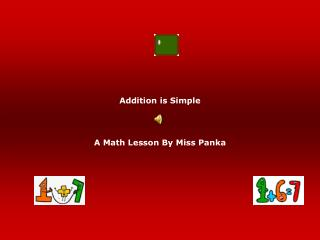# Addition is Simple - PowerPoint PPT PresentationDownload PresentationDownload Presentation- - - - - - - - - - - - - - - - - - - - - - - - - - - E N D - - - - - - - - - - - - - - - - - - - - - - - - - - -
##### Presentation Transcript

1. Addition is Simple A Math Lesson By Miss Panka

2. What is addition? • Addition is when we add two numbers together to find out how many you have in all • We will start out learning addition by counting how many objects there are in total. • When we set up an addition problem we use what is called a plus sign ‘+’. • We will then see how to set up addition problems as another way to see how many objects we have all together.

3. How Many Apples are there? How many apples are there? There is 1 apple. How many apples are there now? There are 2 apples. 1 + 1 = How Many? The answer is 2!!!

4. How many pencils are there? We see 2 pencils. How many pencils are there now? There are now 2 more pencils. How many pencils do we have all together? 2 + 2 = How many? The answer is 4!!

5. How many stars are there? We see 3 stars. Now we see 3 more stars. How many stars do we see all together? 3 stars + 3 more stars = How many? The answer is 6!!

6. How many ice cream cones are there? There are 4 pink ice cream cones. There are 4 green ice cream cones. How many ice cream cones do we all together? 4 pinkice cream cones +4 greenice cream cones = There are 8 ice cream cones all together.

7. How many boxes of crayons do we see? How many boxes of crayons do we see? We see 5 boxes of crayons. There are 5 more boxes of crayons. How many boxes of crayons do we have all together? 5 boxes + 5 more boxes = How many? The answer is 10!!!

8. How many bananas and crayons are there? How many bananas are there? How many crayons do we see? How many bananas and crayons are there all together? 1 banana + 2 crayons = The answer is 3!!!

9. How many watermelons and smiling suns are there? How many watermelons do we see? There are 3 watermelons. How many smiling suns do we see? There is 1 smiling sun. How many watermelons and suns do we see all together? 3 watermelons + 1 smiling sun = The answer is 4!!!

10. How many ice cream cones and strawberries do we see? How many strawberries are there? There are 4 strawberries. How many ice cream cones are there? 1 ice cream cone How many strawberries and ice cream cones do we see all together? 4 strawberries + 1 ice cream cone = ? The answer is 5!!!!

11. How many stars and school buses are there? How many stars do we see? We see 3 stars. How many school buses do we see? There are 2 School Buses How many stars and school buses do we see all together? There are 3 stars and 2 school buses. 3 + 2 = ? The answer is 5.

12. Let’s try and do some simple addition: How many bananas do we see? How many strawberries do we see? How could we set this up using our addition sign? 2 bananas + 4 strawberries = The answer is 6!!!

13. Here is another one for you to try: How many watermelons are there? How many ice cream cones are there? How could we set this up using our addition sign? 5 watermelons + 2 ice cream cones = The answer is 7!!!

14. And here is another one: How many stars and boxes of crayons are there all together? How would we set this up using our addition sign? 3 stars + 6 boxes of crayons = The answer is 9 !!!

15. Now let’s try some on our own: 1 + 1 = 2+ 2 = 3 + 3 = 4 + 4 = 5 + 5 = 2+ 1 = 4 + 1 = 3 + 1 = 2 + 1 = 5 + 1 =

16. Review of our ‘ simple addition’ facts: What is addition? Addition is when we add two or more things together to find out how many we have in all. What is the name of the special sign that we use in addition? A plus sign.

17. Remember to practice your math facts everyday!!!!! MATH IS FUN!!!!